Courses

# Olympiad Test: Alphabet Test - 2

## 20 Questions MCQ Test Science Olympiad Class 6 | Olympiad Test: Alphabet Test - 2

Description
This mock test of Olympiad Test: Alphabet Test - 2 for Class 6 helps you for every Class 6 entrance exam. This contains 20 Multiple Choice Questions for Class 6 Olympiad Test: Alphabet Test - 2 (mcq) to study with solutions a complete question bank. The solved questions answers in this Olympiad Test: Alphabet Test - 2 quiz give you a good mix of easy questions and tough questions. Class 6 students definitely take this Olympiad Test: Alphabet Test - 2 exercise for a better result in the exam. You can find other Olympiad Test: Alphabet Test - 2 extra questions, long questions & short questions for Class 6 on EduRev as well by searching above.
QUESTION: 1

### Arrange the given words in the alphabetical order and choose the one that comes last. Diagonal, Dialogue, Different, Distance.

Solution:

Diagonal, Dialogue, Different, Distance.

QUESTION: 2

### Arrange the given words in the alphabetical order and choose the one that comes last.

Solution:

Transition, Transmit, Temperature, Temple.

QUESTION: 3

### Arrange the given words in the alphabetical order and choose the one that comes last.

Solution:

Waiting, Warring, Watching, Waving.

QUESTION: 4

Directions: In each of the following questions find out which of the letter series follows the given rule.

Q. Number of letters skipped in between adjacent letters in the series is odd.

Solution:

B  C  D  E F G  H  I J K  L  M N O P Q  R
1 3 3 5
1, 3, 5 are all odd numbers.

QUESTION: 5

Directions: In each of the following questions find out which of the letter series follows the given rule.

Q. Number of letters skipped in between adjacent letters in the series is in the order of 2, 5, 7, 10.

Solution:

Q  R S  T  U V W X Y  Z  A B C  D E F G
2  5  7
H  I J K L M N  O P Q R  S
10

QUESTION: 6

Directions: In each of the following questions find out which of the letter series follows the given rule.

Q. Number of letters skipped in between adjacent letters in the series decreases by three.

Solution:

H  I J K L M N O P Q R S T  U  V W X Y Z
12                                                        9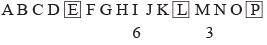QUESTION: 7

Directions: In each of the following questions find out which of the letter series follows the given rule.

Q. Number of letters skipped in between adjacent letters in the series decreases by one each time.

Solution: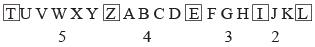QUESTION: 8

Directions: In each of the following questions find out which of the letter series follows the given rule.

Q. Number of letters skipped in between adjacent letters in the series doubles every time.

Solution: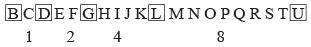QUESTION: 9

Directions: In each of the following questions find out which of the letter series follows the given rule.

Q. Number of letters skipped in between adjacent letters of the series starting from behind increases by one.

Solution: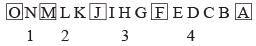QUESTION: 10

Directions: In each of the following questions find out which of the letter series follows the given rule.

Q. Number of letters skipped in between adjacent letters decrease in order.

Solution: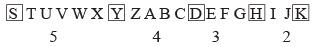QUESTION: 11

Directions: Each of the following questions is based on the following alphabet – series:
A  B  C D E F G H I J K L M N O P Q R S T U V
W X Y Z

Q. Which letter is seventh to the right of the thirteenth letter from the left end?

Solution:

Counting from the left, i.e. from A in the given alphabet series, the thirteenth letter is M. counting from M towards the right, the seventh letter is T.

QUESTION: 12

Directions: Each of the following questions is based on the following alphabet – series:
A  B  C D E F G H I J K L M N O P Q R S T U V
W X Y Z

Q. Which letter in the alphabet is as far from G as T is from M?

Solution:

T is seventh letter to the right of M. Similarly, the seventh letter to the right of G is N.

QUESTION: 13

Directions: Each of the following questions is based on the following alphabet – series:
A  B  C D E F G H I J K L M N O P Q R S T U V
W X Y Z

Q. Which letter is sixteenth to the right of the letter which is fourth to the left of I?

Solution:

The fourth letter to the left of I is E. the sixteenth letter to the right of E is U.

QUESTION: 14

Directions: Each of the following questions is based on the following alphabet – series:
A  B  C D E F G H I J K L M N O P Q R S T U V
W X Y Z

Q. If the order of the English alphabet is reversed, then which letter would be exactly in the middle?

Solution:

The new letter series obtained on reversing the order of the English alphabet is
Z Y X W V U T S R Q P O N M L K J I H G F E D C B A.
Since the series has an even number of letters there is no such letter which lies exactly in the middle.

QUESTION: 15

Directions: Each of the following questions is based on the following alphabet – series:
A  B  C D E F G H I J K L M N O P Q R S T U V
W X Y Z

Q. If only the first half of the given alphabet is reversed, how many letters will be there between K and R?

Solution:

Reversing only the first 13 letters, we obtain the following letter series:
M L K J I H G F E D C B A N O P Q R S T U V W X Y Z.
Clearly, there are 14 letters between K and R in the above series.

QUESTION: 16

Directions: Each of the following questions is based on the following alphabet – series:
A  B  C D E F G H I J K L M N O P Q R S T U V
W X Y Z

Q. If the last ten letters of the alphabet are written in the reverse order, which of the following will be the sixth to the right of the thirteenth letter from the left end?

Solution:

The new alphabet series is:
A B C D E F G H I J K L M N O P Z Y X W V U T S R Q
The thirteenth letter from the left is M. The sixth letter to the right of M is X.

QUESTION: 17

Directions: Each of the following questions is based on the following alphabet – series:
A  B  C D E F G H I J K L M N O P Q R S T U V
W X Y Z

Q. Which letter is exactly midway between G and Q in the given alphabet?

Solution:

There are nine letters between G and Q – H, I, J, K, L, M, N, O, P. Clearly, the middle letter is L.

QUESTION: 18

Directions: Each of the following questions is based on the following alphabet – series:
A  B  C D E F G H I J K L M N O P Q R S T U V
W X Y Z

Q. If 1st and 26th, 2nd and 25th, 3rd and 24th and so on, letters of the English alphabet are paired, then which of the following pair is correct?

Solution:

The pairing up of letters may be done as shown.
AZ, BY, CX, DW, EV, FU, GT, HS, IR, JQ, KP, LO, MN.

QUESTION: 19

Directions: Each of the following questions is based on the following alphabet – series:
A  B  C D E F G H I J K L M N O P Q R S T U V
W X Y Z

Q. If in the English alphabet every fourth letter is replaced by the symbol ( ∗), which of the following would be seventh to the left of the fourteenth element from the left?

Solution:

The new series becomes
A B C ∗ E F G ∗ I J K ∗ M N O ∗ Q R S ∗ U V W ∗ YZ.

QUESTION: 20

Directions: Each of the following questions is based on the following alphabet – series:
A  B  C D E F G H I J K L M N O P Q R S T U V
W X Y Z

Q. In the following alphabets, which letter is eighth to the right of the fourteenth letter from the right end?
Z A B C D E F G H I J K L M N O P Q R S T U V W X Y

Solution:

The fourteenth letter from the right is L. the eighth letter to the right of L is T.